×

Search anything:

# Multilayer Perceptron

#### Machine Learning (ML) Artificial Intelligence perceptronMultilayer perceptron is a fundamental concept in Machine Learning (ML) that lead to the first successful ML model, Artificial Neural Network (ANN). We have explored the idea of Multilayer Perceptron in depth.

# Introduction

We are living in the age of Artificial Intelligence. AI is everywhere, every top company be it technical or financial are trying to achieve excellence in this bleeding-edge technology. According to the World Economic Forum, AI will lead to an increase jobs by 58 million globally ! Studying about Artificial Intelligence may be one of the best self-investment right now. In this blog we'll be discussing the core math behind Aritificial Intelligence or Artificical Neural Network - the Perceptron Model

# Background

The aim of Artificial Intelligence is to mimic the human intelligence as efficiently as possible.An artificial neural network (ANN) is the piece of a computing system designed to simulate the way the human brain analyzes and processes information. Before discussing about ANN let's talk about the perceptron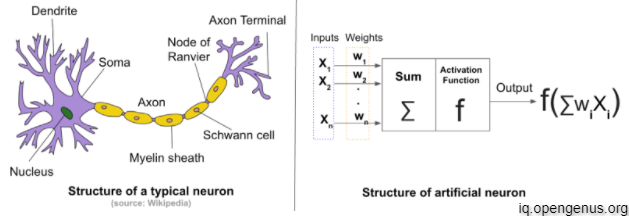# Perceptron

Frank Rosenblatt, an American psychologist, proposed the classical perceptron model in 1958. Further refined and carefully analyzed by Minsky and Papert (1969) — their model is referred to as the perceptron model. A Perceptron is generally used for Binary Classification problems. A Perceptron consists of various inputs, for each input there is a weight and bias. The output of the Perceptron is the biases added to the dot-product of the input with weights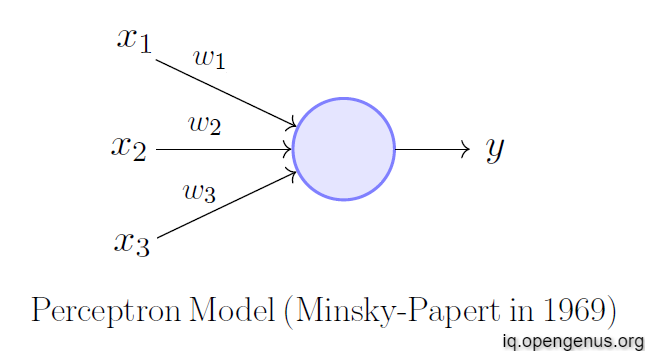In Linear Algebra the output will be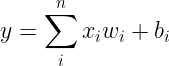Based on the output y we can apply classification and we can adjust the weights and biases to achieve desired results.

Perceptron Model works similar to logic gates to acheive desired results

# How perceptron works?

Let us suppose we want to predict whether we can go to play or not. Following will be our inputs :

• Parent's permission(x1)
• raining outside or not(x2)
• number of players available (x3)

We will provide probabilites of the first two events and the number of players. We know that parent's permission is a must, therefore we'll assign a higher weight to it than others but we'll add a positive bias because we are eager to play. Similarly weights and biases of other events can also be assigned. Let's define our perceptron output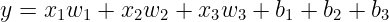A simple perceptron model can be :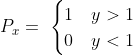Here by 1 and 0 we mean Yes and No respectively !
The same logic can be applied to complex situations with thousands of inputs

# Artificial Neural Networks

ANN is the foundation of artificial intelligence (AI) and solves problems that would prove impossible or difficult by human or statistical standards. Above we saw simple single perceptron. When more than one perceptrons are combined to create a dense layer where each output of the previous layer acts as an input for the next layer it is called a Multilayer Perceptron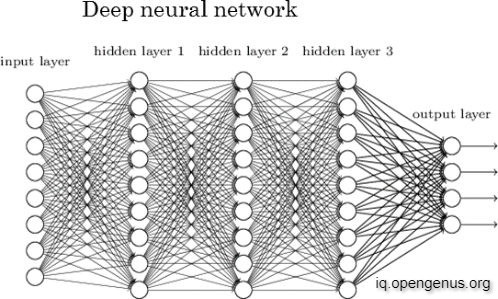An ANN slightly differs from the Perceptron Model. Instead of just simply using the output of the perceptron, we apply an Activation Function to the perceptron's output. When an activation function is applied to a Perceptron, it is called a Neuron. The combination of different layers of neurons connected to each other is called a Neural Network.The activation function decides whether a certain neuron must be fired or not. Some common activation functions are the sigmoid function and the Rectified Linear Unit (ReLU).

Sigmoid Function: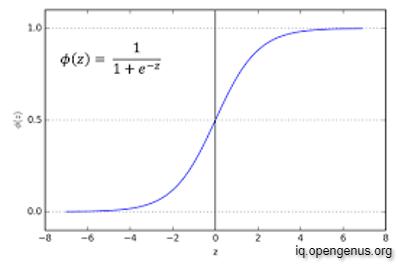ReLU Function :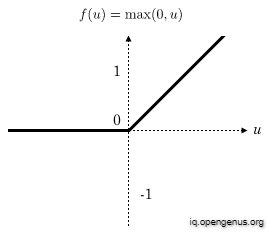# Terminologies in Neural Network

## Input Layer

The first layer of our ANN is called the input layers. This layer takes input of our data which is then multiplied with weights and bais added is forwarded to the next layers

## Output Layer

The last layer of the neural network is called the Output layer. In case of Regression problem, the output layer consists of only one neuron. In case of Classification problem, our Output layer may consist of different neurons based on the loss function.

## Hidden Layers

The layers between the input and output layers are called the hidden layers. There may be hundreds of hidden layers based on the complexity of the data and problem we are solving

## Loss Function

Neural networks are trained using an optimization process that requires a loss function to calculate the model error.In case of Regression the most commonly used loss function is the Mean Squared Error.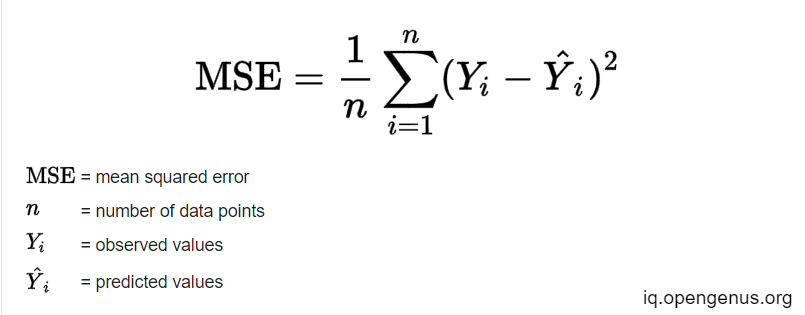For Classification a popular loss function is Cross-Entropy Loss. Since, heavy math is involved here, all these functions deserve an article themselves.

## Backpropogation

Backpropogation is an efficient method used in neural networks to find the gradient of the error with respect to the weights and biases. The "backwards" part of the name stems from the fact that calculation of the gradient proceeds backwards through the network, with the gradient of the final layer of weights being calculated first and the gradient of the first layer of weights being calculated last. Partial computations of the gradient from one layer are reused in the computation of the gradient for the previous layer. This backwards flow of the error information allows for efficient computation of the gradient at each layer versus the naive approach of calculating the gradient of each layer separately.

Again, Backpropogation is a heavy topic itself, so this will do for now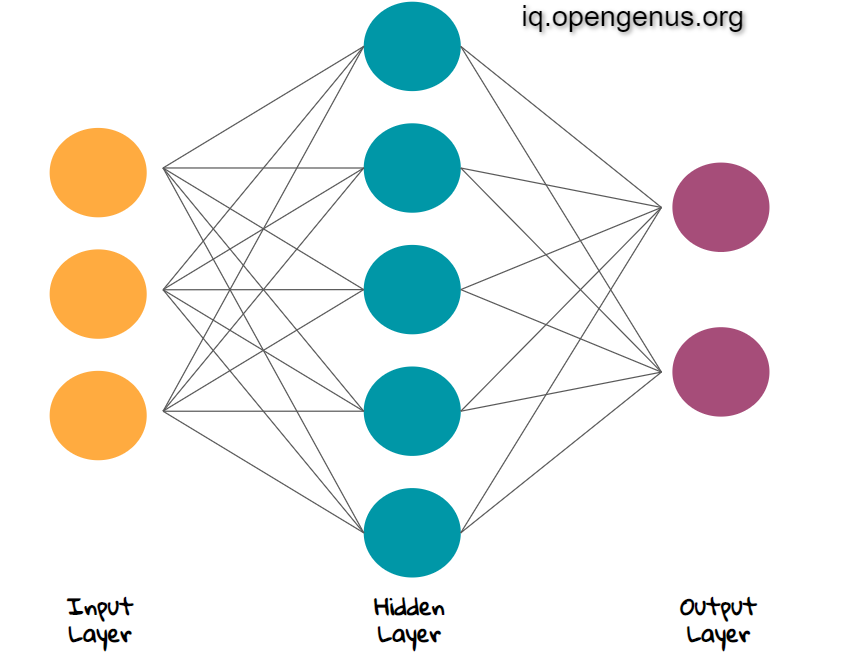# Conclusion

In this blog we saw the core logic behind Artificial Intelligence - Neural Networks and Multilayer Perceptrons. AI is a vast topic and it is difficult to explain all the topics at once. Check out other OpenGenus Articles about Artificial Intelligence